## Project Phases:

• 1) Exploratory Analysis and Data Cleaning
• 2) Feature Importance
• 3) Train Model
• 4) Voting
• 5) Submission

import pandas as pd
import numpy as np
from matplotlib import pyplot as plt
import seaborn as sns
from scipy import stats
from matplotlib.gridspec import GridSpec
from scipy.special import boxcox1p
import warnings

from sklearn.ensemble import GradientBoostingClassifier, ExtraTreesClassifier, VotingClassifier
from sklearn.model_selection import GridSearchCV
from sklearn.tree import DecisionTreeClassifier
from sklearn.svm import SVC
from xgboost import XGBClassifier

warnings.filterwarnings("ignore") # ignoring annoying warnings


Ignoring warnings that are not relevant for this project.

warnings.filterwarnings("ignore")


gender_submission = pd.read_csv("../input/titanic/gender_submission.csv")
test["Survived"] = np.nan # we don't have target values for the test


Here we concat train and test into one, so we can analyze everything and replace nan values later based on all dataset.

dataset = pd.concat([train,test],axis=0).reset_index(drop=True)
dataset = dataset.fillna(np.nan)


# 1-Exploratory Analysis and Data Cleaning

dataset.head(10)

Age Cabin Embarked Fare Name Parch PassengerId Pclass Sex SibSp Survived Ticket
0 22.0 NaN S 7.2500 Braund, Mr. Owen Harris 0 1 3 male 1 0.0 A/5 21171
1 38.0 C85 C 71.2833 Cumings, Mrs. John Bradley (Florence Briggs Th... 0 2 1 female 1 1.0 PC 17599
2 26.0 NaN S 7.9250 Heikkinen, Miss. Laina 0 3 3 female 0 1.0 STON/O2. 3101282
3 35.0 C123 S 53.1000 Futrelle, Mrs. Jacques Heath (Lily May Peel) 0 4 1 female 1 1.0 113803
4 35.0 NaN S 8.0500 Allen, Mr. William Henry 0 5 3 male 0 0.0 373450
5 NaN NaN Q 8.4583 Moran, Mr. James 0 6 3 male 0 0.0 330877
6 54.0 E46 S 51.8625 McCarthy, Mr. Timothy J 0 7 1 male 0 0.0 17463
7 2.0 NaN S 21.0750 Palsson, Master. Gosta Leonard 1 8 3 male 3 0.0 349909
8 27.0 NaN S 11.1333 Johnson, Mrs. Oscar W (Elisabeth Vilhelmina Berg) 2 9 3 female 0 1.0 347742
9 14.0 NaN C 30.0708 Nasser, Mrs. Nicholas (Adele Achem) 0 10 2 female 1 1.0 237736

Let's see the dataset types, nan quantity for each column and describe them.

dataset.dtypes

Age            float64
Cabin           object
Embarked        object
Fare           float64
Name            object
Parch            int64
PassengerId      int64
Pclass           int64
Sex             object
SibSp            int64
Survived       float64
Ticket          object
dtype: object
dataset.isnull().sum(axis = 0)

Age             263
Cabin          1014
Embarked          2
Fare              1
Name              0
Parch             0
PassengerId       0
Pclass            0
Sex               0
SibSp             0
Survived        418
Ticket            0
dtype: int64

Age, Cabin, Fare and Embarked have nan values. We will need to analyze each feature individually to get better results. Survived has nan values just because of test data.

dataset.describe()

Age Fare Parch PassengerId Pclass SibSp Survived
count 1046.000000 1308.000000 1309.000000 1309.000000 1309.000000 1309.000000 891.000000
mean 29.881138 33.295479 0.385027 655.000000 2.294882 0.498854 0.383838
std 14.413493 51.758668 0.865560 378.020061 0.837836 1.041658 0.486592
min 0.170000 0.000000 0.000000 1.000000 1.000000 0.000000 0.000000
25% 21.000000 7.895800 0.000000 328.000000 2.000000 0.000000 0.000000
50% 28.000000 14.454200 0.000000 655.000000 3.000000 0.000000 0.000000
75% 39.000000 31.275000 0.000000 982.000000 3.000000 1.000000 1.000000
max 80.000000 512.329200 9.000000 1309.000000 3.000000 8.000000 1.000000

This results shows that probably Fare, for example, has outliers, since its maximum value is so much higher than 75% of the data. Also the mean is very different from median (50%).

### SibSp and Parch

sns.factorplot(x='SibSp',
size= 7,
aspect= 2,
data=train,
y ='Survived',
palette = "dark")
plt.xlabel('\nSibSp')
plt.ylabel('Survival Probability\n')
plt.show()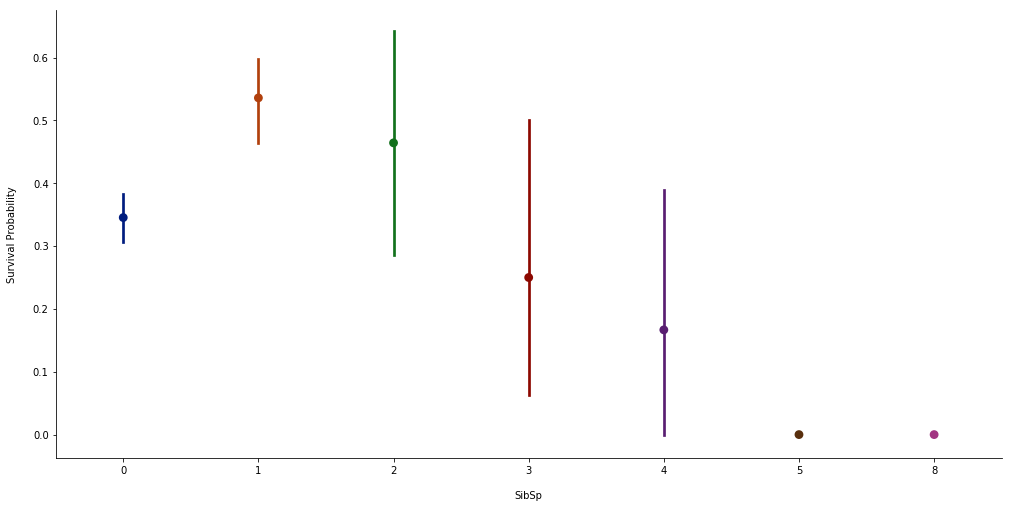Small numbers of SibSp have higher probability to survive.

sns.factorplot(x='Parch',
size= 7,
aspect= 2,
data=train,
y ='Survived',
palette = "dark")
plt.xlabel('\nParch')
plt.ylabel('Survival Probability\n')
plt.show()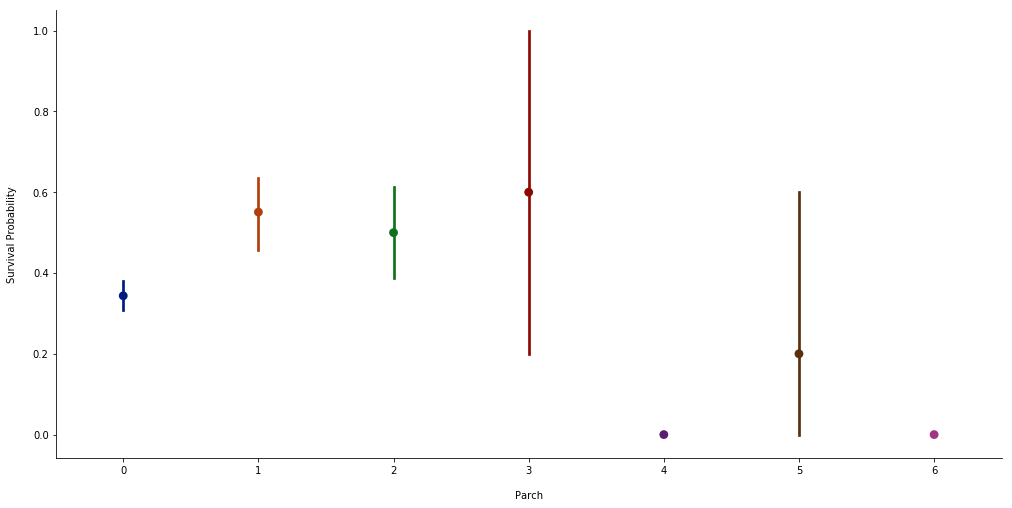Small numbers of Parch have higher probability to survive.

Since these features have similar behavior, then we can add them with each person being analyzed.

dataset["Family"] = dataset["SibSp"] + dataset["Parch"] + 1
train["Family"] = train["SibSp"] + train["Parch"] + 1
test["Family"] = test["SibSp"] + test["Parch"] + 1


Repeating the factorplot for the Family.

sns.factorplot(x='Family',
size= 7,
aspect= 2,
data=train,
y ='Survived',
palette = "dark")
plt.xlabel('\nFamily')
plt.ylabel('Survival Probability\n')
plt.show()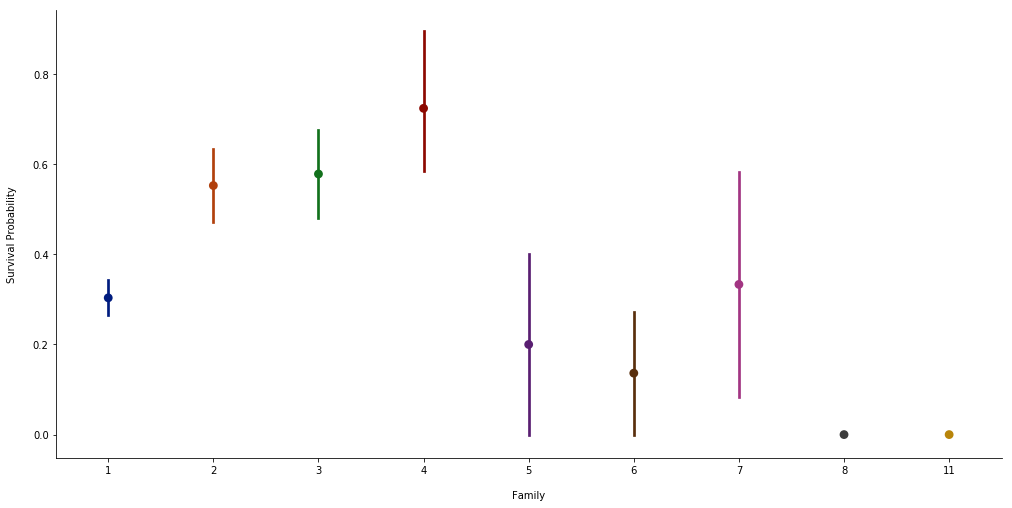we don't need these features anymore, since we created another one.

dataset = dataset.drop(columns=["SibSp","Parch"])
train = train.drop(columns=["SibSp","Parch"])
test = test.drop(columns=["SibSp","Parch"])

plt.figure(figsize=(15,8))
sns.countplot(data=train,
x='Family',
palette = "dark")
plt.xlabel('\nFamily')
plt.ylabel('Number of Occurrences\n')
plt.show()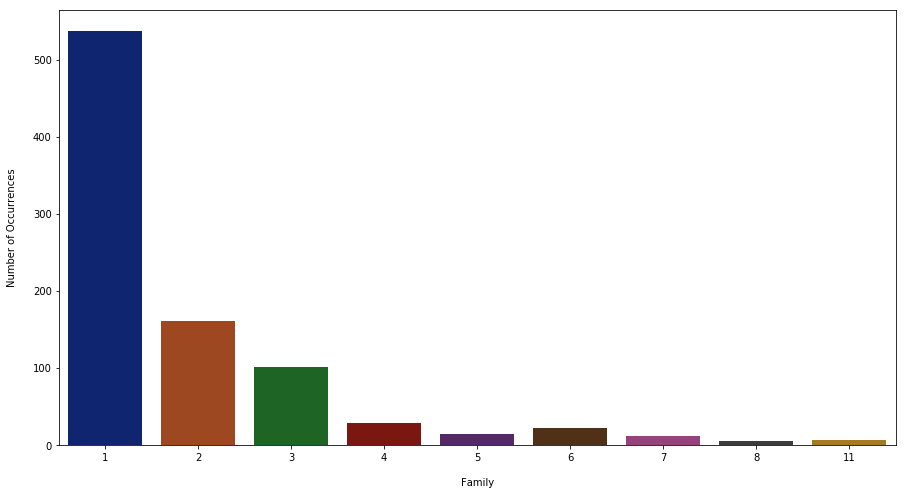The countplot shows that the more families grow, the fewer occurrences happen.

Since there are 11 different categories for Family, lets group them in single, medium and big families.

dataset.Family = list(map(lambda x: 'Big' if x > 4 else('Single' if x == 1 else 'Medium'), dataset.Family))
train.Family = list(map(lambda x: 'Big' if x > 4 else('Single' if x == 1 else 'Medium'), train.Family))
test.Family = list(map(lambda x: 'Big' if x > 4 else('Single' if x == 1 else 'Medium'), test.Family))

plt.figure(figsize=(15,8))
sns.countplot(data=train,
x='Family',
palette = "dark")
plt.xlabel('\nFamily')
plt.ylabel('Number of Occurrences\n')
plt.show()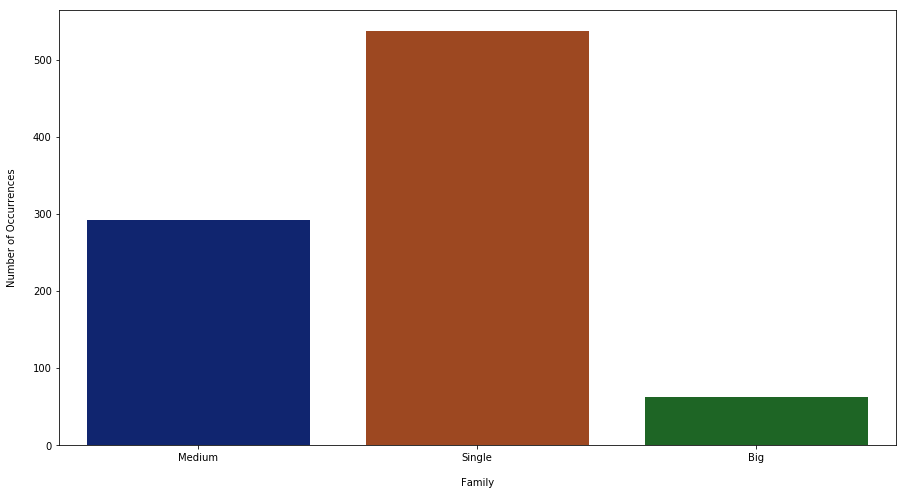Now we have 3 categories.

sns.factorplot(x='Family',
size= 7,
aspect= 2,
data=train,
y ='Survived',
palette = "dark")
plt.xlabel('\nFamily')
plt.ylabel('Survival Probability\n')
plt.show()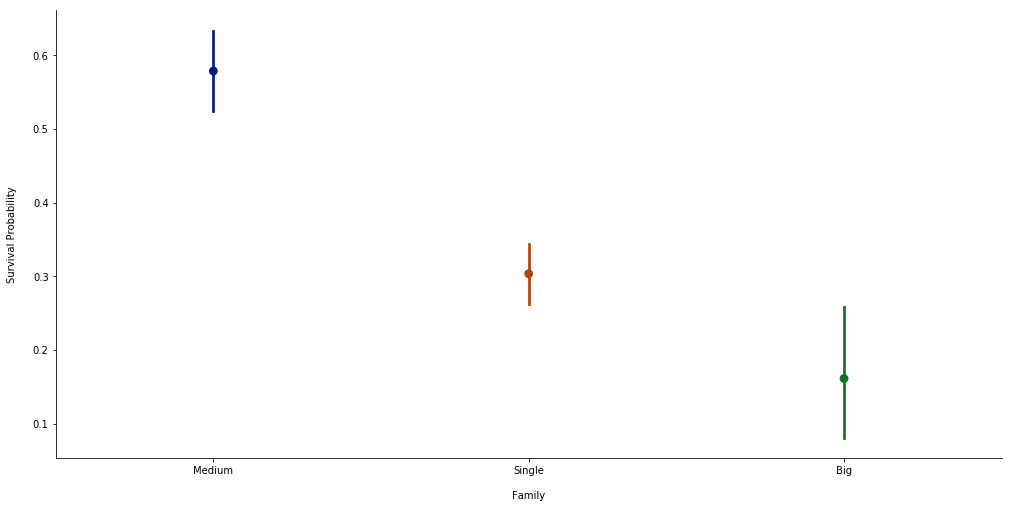### Sex

sns.factorplot(x='Sex',
size= 7,
aspect= 2,
data=train,
y ='Survived',
palette = "dark")
plt.xlabel('\nSex')
plt.ylabel('Survival Probability\n')
plt.show()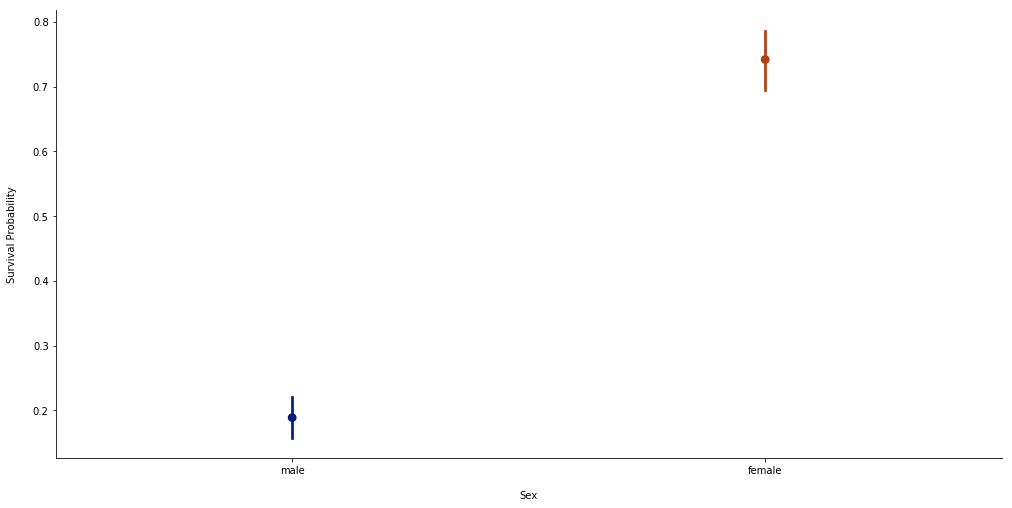This clearly shows that a woman has higher probability to survive.

Many models need to receive numbers, not text. So, let's change sex from string to integer type.

dataset.Sex = dataset.Sex.map({'male': 0, 'female': 1})
train.Sex = train.Sex.map({'male': 0, 'female': 1})
test.Sex = test.Sex.map({'male': 0, 'female': 1})


### Pclass

sns.factorplot(x='Pclass',
size= 7,
aspect= 2,
data=train,
y ='Survived',
kind='bar',
palette = "dark")
plt.xlabel('\nPclass')
plt.ylabel('Survival Probability\n')
plt.show()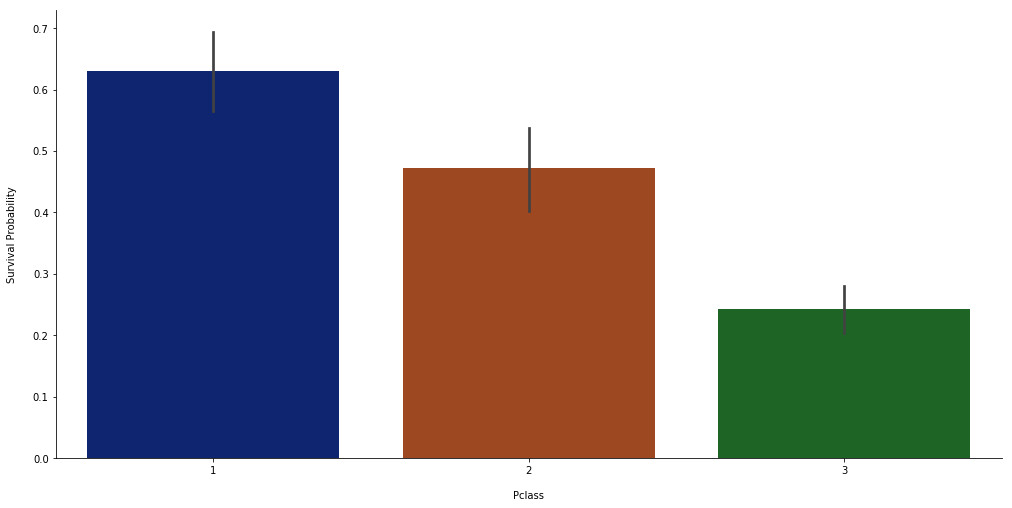This shows that first class has higher probability to survive, probably because of influence.

### Fare

Since we have not so many nan values for this feature, then we can use the dataset median to fill them.

dataset["Fare"] = dataset["Fare"].fillna(dataset["Fare"].median())
train["Fare"] = train["Fare"].fillna(dataset["Fare"].median())
test["Fare"] = test["Fare"].fillna(dataset["Fare"].median())


Fare can be considered as continuous variable, so we can plot its distribution.

plt.figure(figsize=(20,8))
sns.distplot(train['Fare'], color = "steelblue", hist_kws={"rwidth":0.80, 'alpha':1.0})
plt.xticks(np.arange(0,600,10),rotation=45)
plt.xlabel('\nFare')
plt.ylabel('Distribution\n')
plt.show()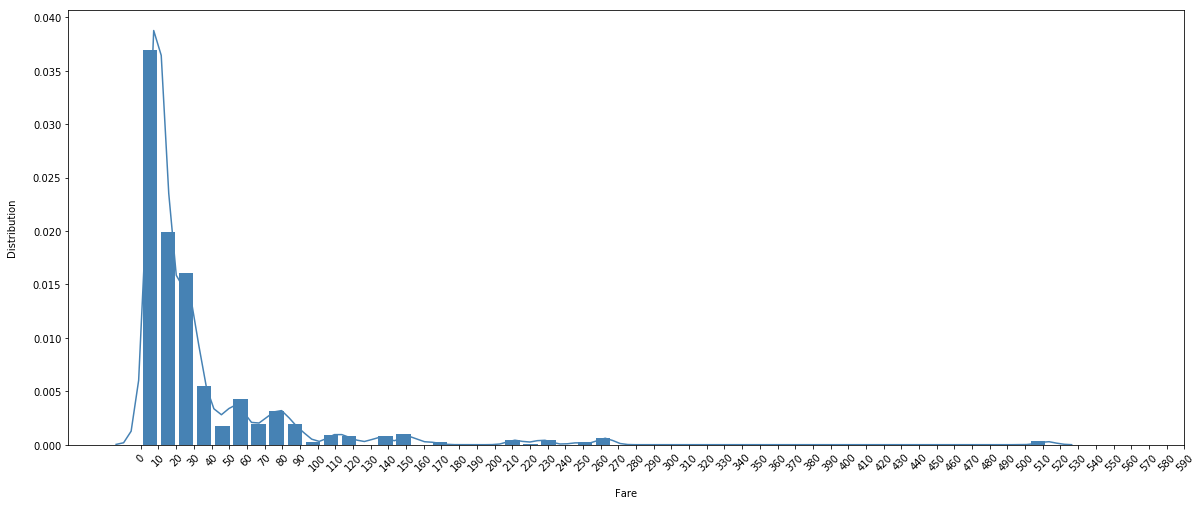It seems that the curve has a positive skewness (to the left).

plt.figure(figsize=(15,8))
sns.violinplot(y='Fare',
data=dataset,
x='Survived',
palette = "dark")
plt.xlabel('\nSurvived')
plt.ylabel('Fare\n')
plt.show()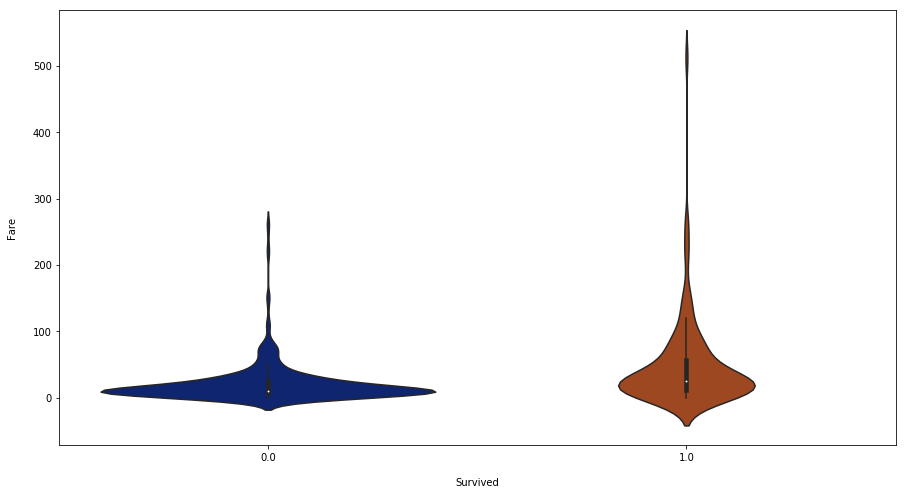Probably the people who payed more have higher probability to suvive, but there aren't many.

Let's divide Fare in categories, first we need to create balanced category shapes.

dataset[dataset.Fare.between(0,10)].shape

(491, 11)
dataset[dataset.Fare.between(11,25)].shape

(291, 11)
dataset[dataset.Fare.between(26,50)].shape

(236, 11)
dataset[dataset.Fare > 51].shape

(238, 11)

So, lets divide the feature values into 3 categories, with similiar shape.

dataset.Fare = list(map(lambda x: 'Very Low' if x <= 10
else('Low' if (x > 10 and x < 26)
else('Medium' if (x >= 26 and x <= 50) else 'High')), dataset.Fare))

train.Fare = list(map(lambda x: 'Very Low' if x <= 10
else('Low' if (x > 10 and x < 26)
else('Medium' if (x >= 26 and x <= 50) else 'High')), train.Fare))

test.Fare = list(map(lambda x: 'Very Low' if x <= 10
else('Low' if (x > 10 and x < 26)
else('Medium' if (x >= 26 and x <= 50) else 'High')), test.Fare))

sns.factorplot(x='Fare',
size= 7,
aspect= 2,
data=train,
y ='Survived',
palette = "dark")
plt.xlabel('\nFare')
plt.ylabel('Survival Probability\n')
plt.show()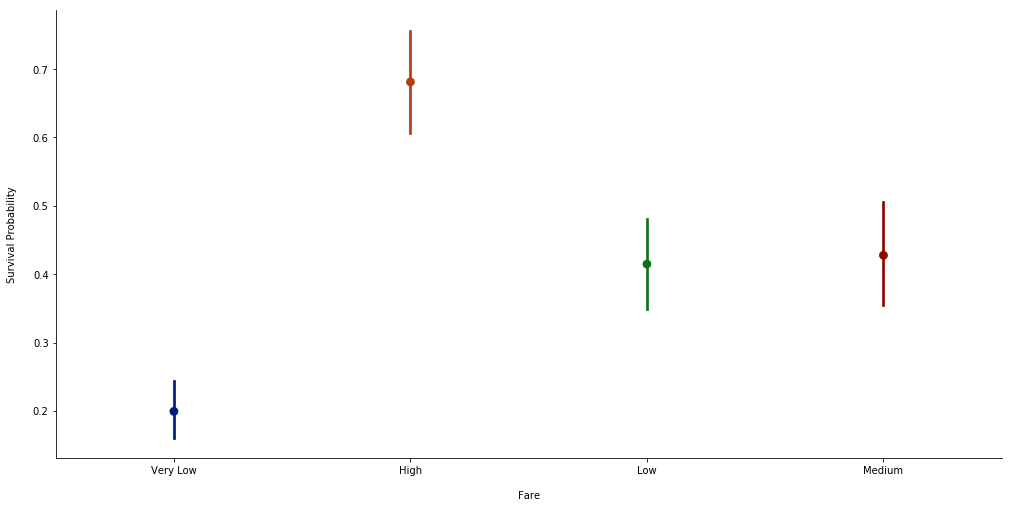Here we can see that high fare people have higher probability to survive, and very low fare people have not.

### Embarked

plt.figure(figsize=(15,8))
sns.countplot(x='Embarked',
data=train,
palette = "dark")
plt.xlabel('\nEmbarked')
plt.ylabel('Number of Occurrences\n')
plt.show()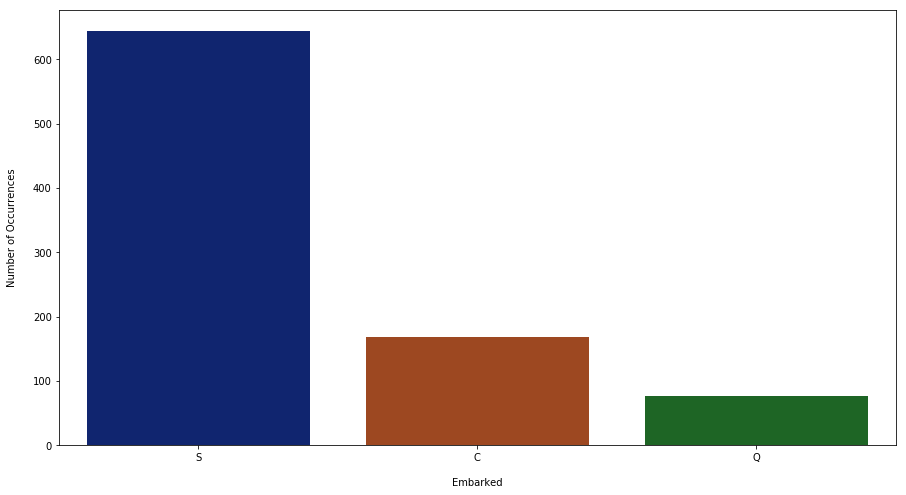'S' ir more frequent in the dataset.

sns.factorplot(x='Embarked',
size= 7,
aspect= 2,
data=train,
y ='Survived',
palette = "dark")
plt.xlabel('\nEmbarked')
plt.ylabel('Survival Probability\n')
plt.show()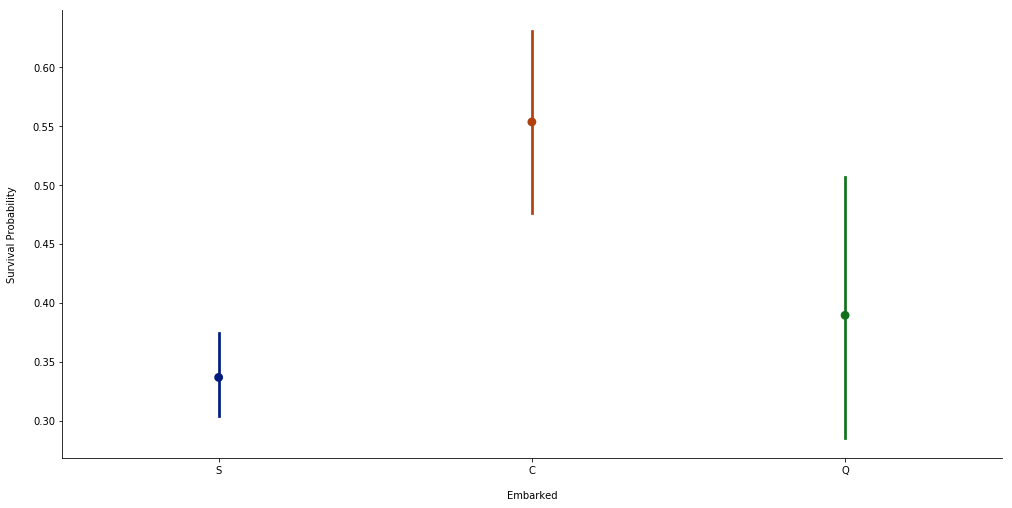Another variable that shows a difference in survival probability.

We are going to fill the two nan values with the most frequent category.

dataset.Embarked = dataset.Embarked.fillna('S')
train.Embarked = train.Embarked.fillna('S')
test.Embarked = test.Embarked.fillna('S')


### Name

Getting the title (Mr, Mrs, Miss and others) which is present in all rows and creating another column.

title = []
for i in dataset.Name.str.split(', '):
title.append(i.split('. '))
dataset["Title"] = title

title = []
for i in train.Name.str.split(', '):
title.append(i.split('. '))
train["Title"] = title

title = []
for i in test.Name.str.split(', '):
title.append(i.split('. '))
test["Title"] = title


Dropping Name column that we don't need anymore.

dataset = dataset.drop(columns=["Name"])
train = train.drop(columns=["Name"])
test = test.drop(columns=["Name"])

plt.figure(figsize=(20,8))
sns.countplot(dataset.Title, palette = "dark")
plt.xticks(rotation=45)
plt.xlabel('\nTitle')
plt.ylabel('Number of Occurrences\n')
plt.show()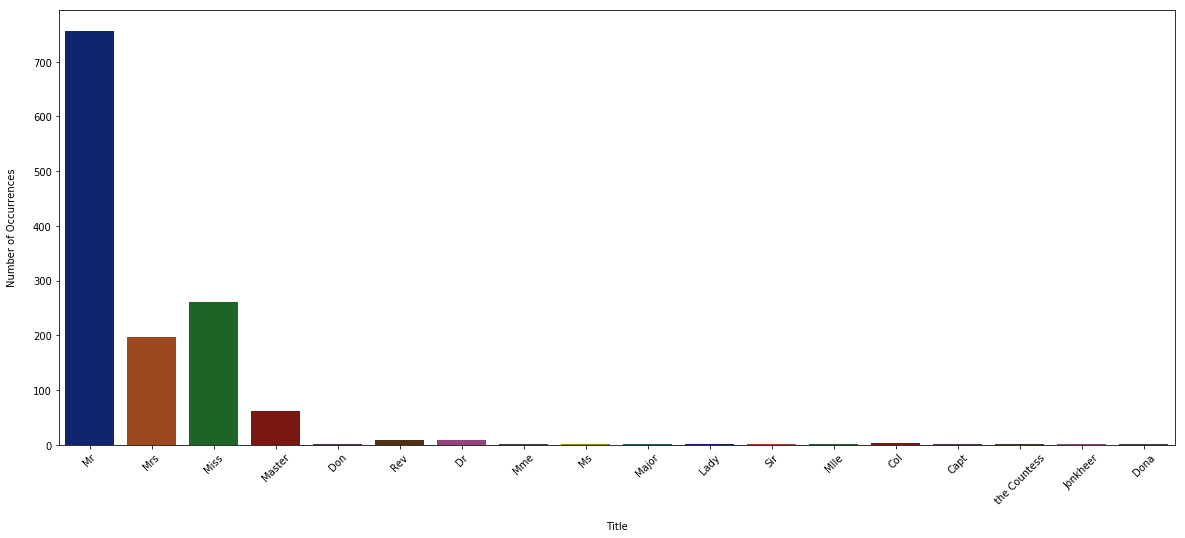Titles frequency: we can see that the majority of the people has titles like 'Mr', 'Mrs' and 'Miss'. We can group the others into one category.

dataset.Title = list(map(lambda x: x if (x == 'Mr' or x == 'Mrs' or x == 'Miss')
else('Other'), dataset.Title))

train.Title = list(map(lambda x: x if (x == 'Mr' or x == 'Mrs' or x == 'Miss')
else('Other'), train.Title))

test.Title = list(map(lambda x: x if (x == 'Mr' or x == 'Mrs' or x == 'Miss')
else 'Other', test.Title))

plt.figure(figsize=(15,8))
sns.countplot(dataset.Title, palette = "dark")
plt.xlabel('\nTitle')
plt.ylabel('Number of Occurrences\n')
plt.show()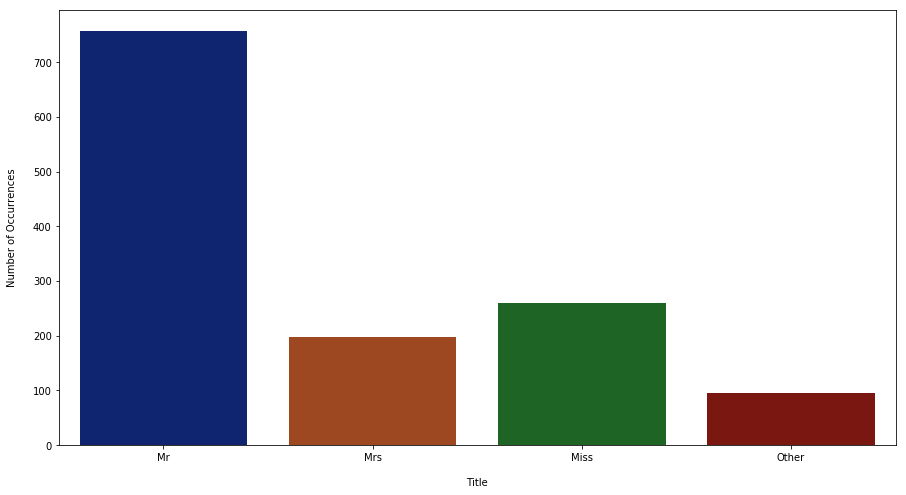sns.factorplot(x='Title',
size= 7,
aspect= 2,
data=train,
y ='Survived',
palette = "dark")
plt.xlabel('\nTitle')
plt.ylabel('Survival Probability\n')
plt.show()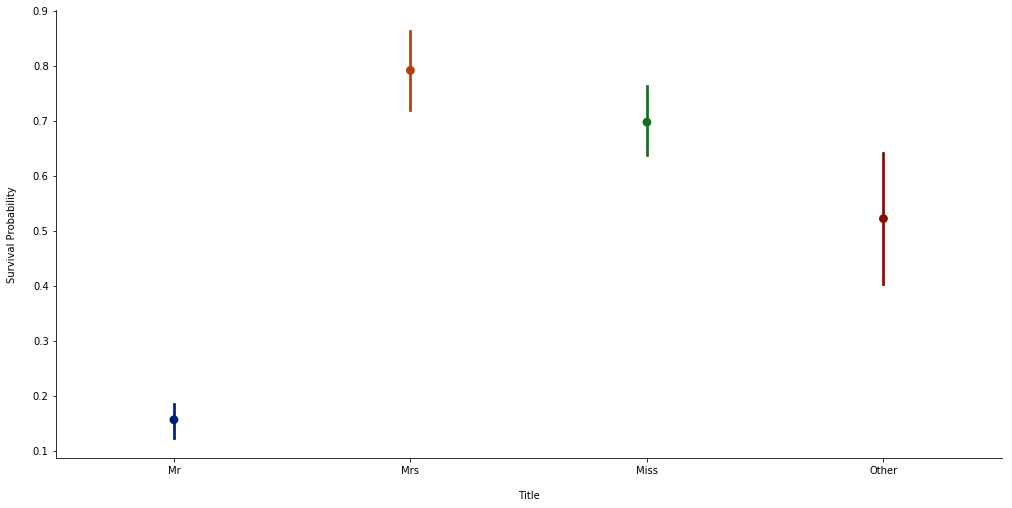Clearly the gentlemen are in danger.

### Cabin

This variable doesn't seem to have a lot of value except the first letter. So let's extract it, if nan then let the letter be 'Z'.

cabin = []
for i in dataset.Cabin:
if type(i) != float:
cabin.append(i)
else:
cabin.append('Z')
dataset.Cabin = cabin

cabin = []
for i in train.Cabin:
if type(i) != float:
cabin.append(i)
else:
cabin.append('Z')
train.Cabin = cabin

cabin = []
for i in test.Cabin:
if type(i) != float:
cabin.append(i)
else:
cabin.append('Z')
test.Cabin = cabin

plt.figure(figsize=(15,8))
sns.countplot(dataset.Cabin, palette = "dark")
plt.xlabel('\nCabin')
plt.ylabel('Number of Occurrences\n')
plt.show()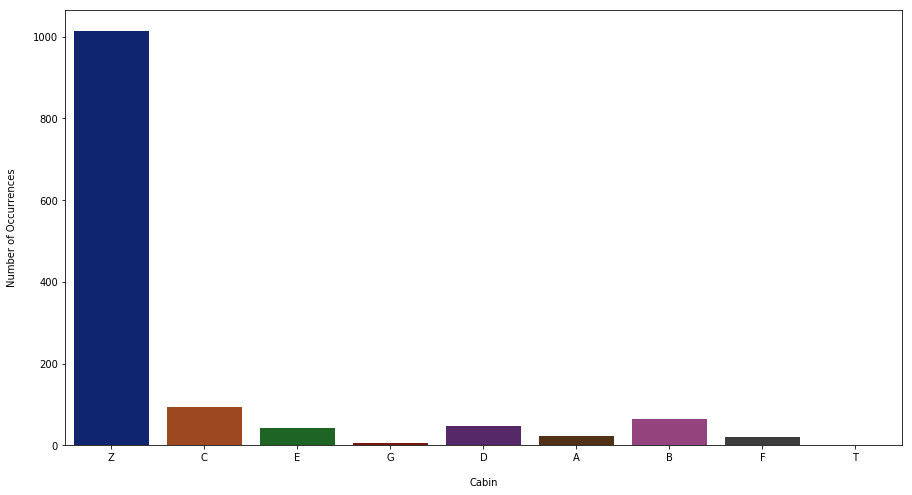sns.factorplot(x='Cabin',
size= 7,
aspect= 2,
data=train,
y ='Survived',
palette = "dark")
plt.xlabel('\nCabin')
plt.ylabel('Survival Probability\n')
plt.show()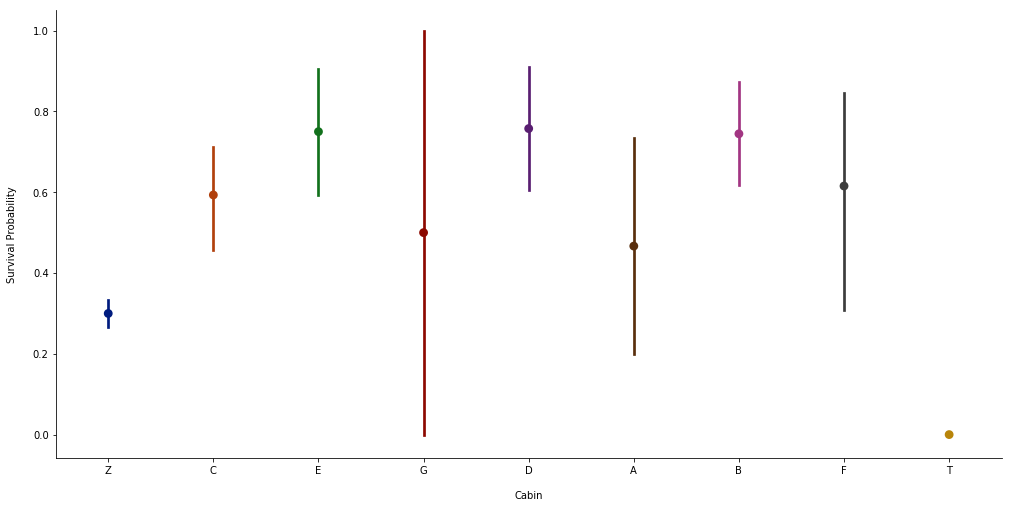It seems that people without cabines have less chance to survive, but standard deviations are large for some letters. We can group letters with similar behaviors.

dataset.Cabin = dataset.Cabin.map({'B':'BCDE','C':'BCDE','D':'BCDE','E':'BCDE','A':'AFG','F':'AFG','G':'AFG','Z':'Z','T':'Z'})
train.Cabin = train.Cabin.map({'B':'BCDE','C':'BCDE','D':'BCDE','E':'BCDE','A':'AFG','F':'AFG','G':'AFG','Z':'Z','T':'Z'})
test.Cabin = test.Cabin.map({'B':'BCDE','C':'BCDE','D':'BCDE','E':'BCDE','A':'AFG','F':'AFG','G':'AFG','Z':'Z','T':'Z'})


Counting them again, by group.

plt.figure(figsize=(15,8))
sns.countplot(dataset.Cabin, palette = "dark")
plt.xlabel('\nCabin')
plt.ylabel('Number of Occurrences\n')
plt.show()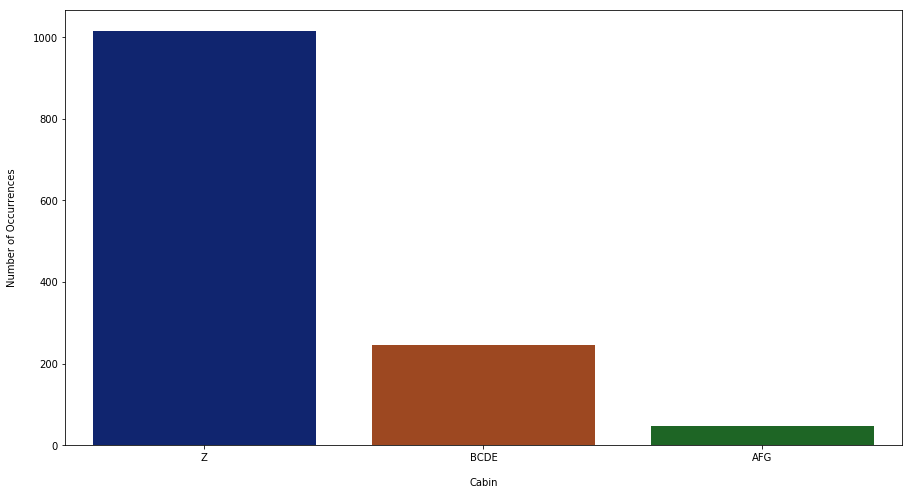New factorplot.

sns.factorplot(x='Cabin',
size= 7,
aspect= 2,
data=train,
y ='Survived',
palette = "dark")
plt.xlabel('\nCabin')
plt.ylabel('Survival Probability\n')
plt.show()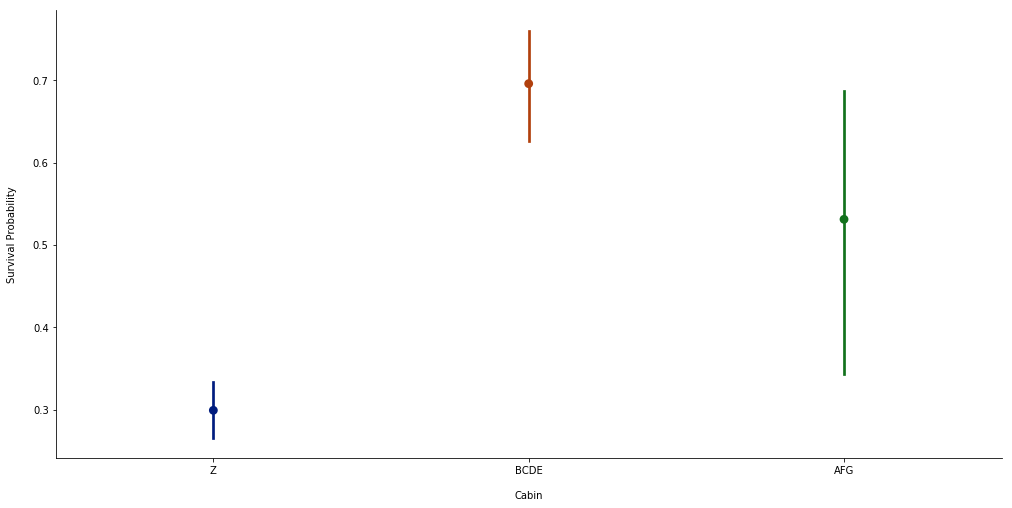### Ticket

This variable also doesn't seem to have a lot of value except the first number.

tickets = []
for i in dataset.Ticket:
tickets.append(i.split(' ')[-1])
dataset.Ticket = tickets

tickets = []
for i in train.Ticket:
tickets.append(i.split(' ')[-1])
train.Ticket = tickets

tickets = []
for i in test.Ticket:
tickets.append(i.split(' ')[-1])
test.Ticket = tickets


Let's see the number of occurrences for each number.

plt.figure(figsize=(15,8))
sns.countplot(dataset.Ticket.sort_values(), palette = "dark")
plt.xlabel('\nTicket')
plt.ylabel('Number of Occurrences\n')
plt.show()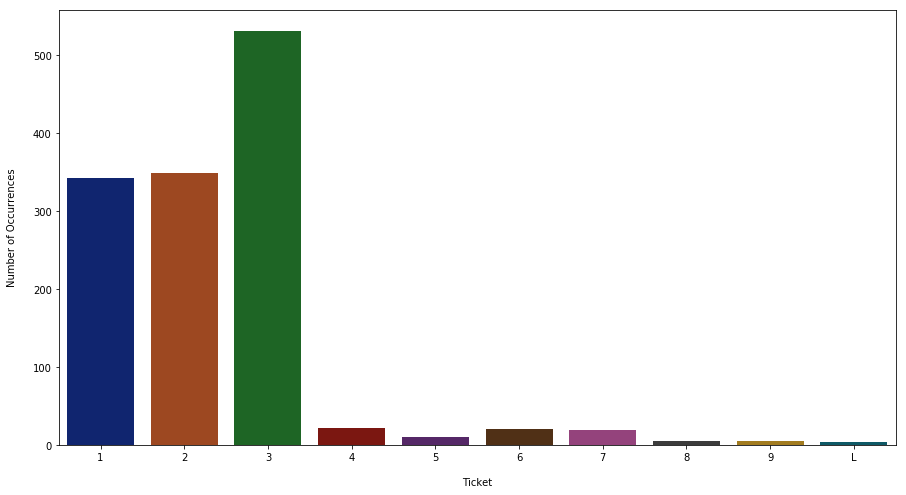The more frequent numbers are 1, 2 and 3.

sns.factorplot(x='Ticket',
size= 7,
aspect= 2,
data=train,
y ='Survived',
palette = "dark",
order=train.Ticket.sort_values().unique())
plt.xlabel('\nTicket')
plt.ylabel('Survival Probability\n')
plt.show()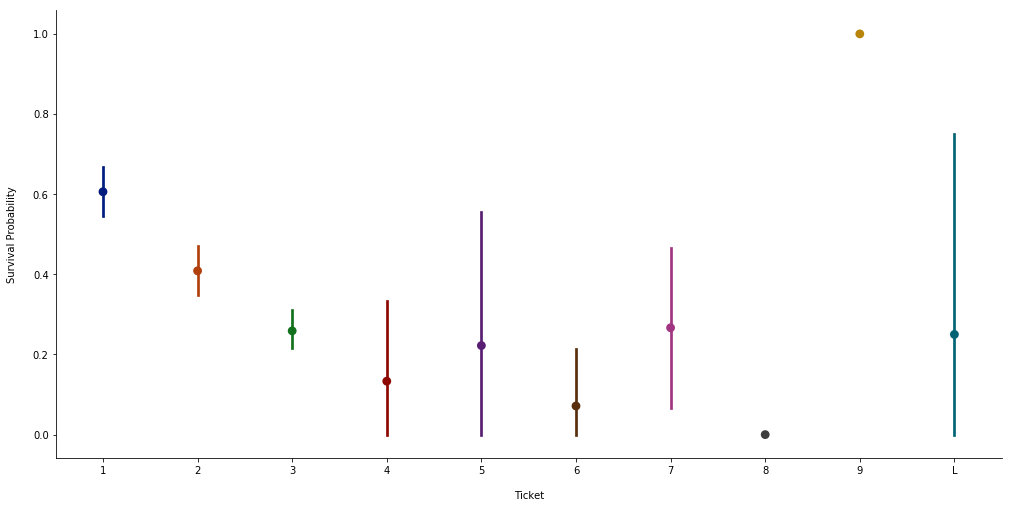Since 1, 2 and 3 have more occurrences and the others have large standard deviations, we can group these into one category.

dataset.Ticket = list(map(lambda x: 4 if (x == 'L' or int(x) >= 4) else int(x), dataset.Ticket))
train.Ticket = list(map(lambda x: 4 if (x == 'L' or int(x) >= 4) else int(x), train.Ticket))
test.Ticket = list(map(lambda x: 4 if (x == 'L' or int(x) >= 4) else int(x), test.Ticket))


New countplot.

plt.figure(figsize=(15,8))
sns.countplot(dataset.Ticket.sort_values(), palette = "dark")
plt.xlabel('\nTicket')
plt.ylabel('Number of Occurrences\n')
plt.show()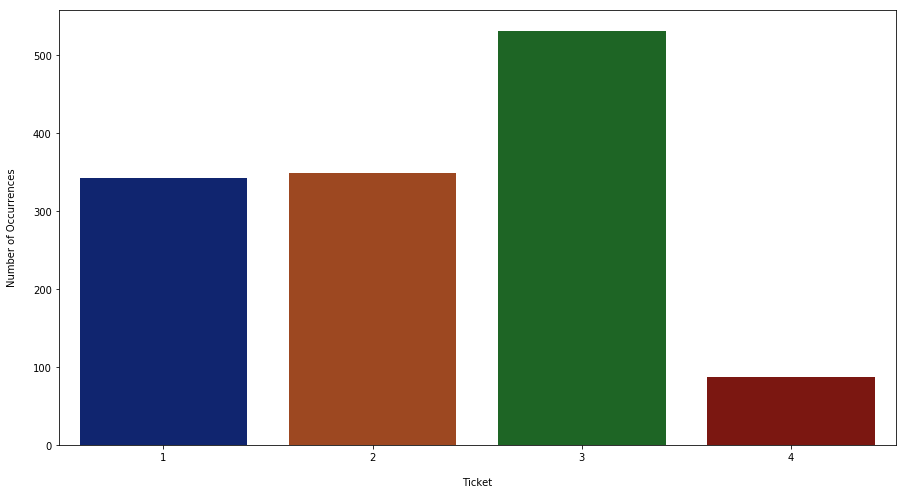And now we have 4 categories, with different probabilities.

sns.factorplot(x='Ticket',
size= 7,
aspect= 2,
data=train,
y ='Survived',
palette = "dark",
order=train.Ticket.sort_values().unique())
plt.xlabel('\nTicket')
plt.ylabel('Survival Probability\n')
plt.show()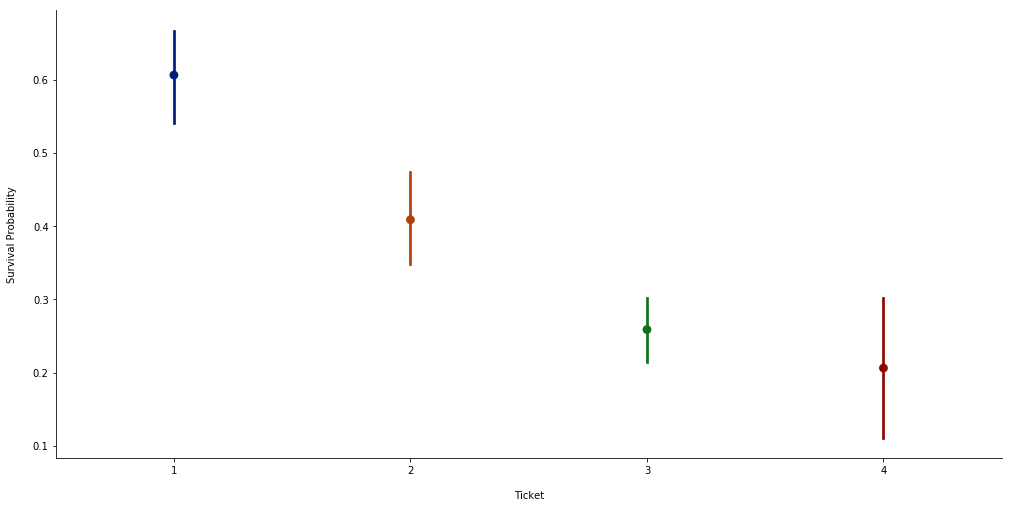### Age

We will need to see which features have more correlation with age, so we can safely replace nan values.

plt.figure(figsize=(15,8))
sns.boxplot(x='Family', data=dataset,y='Age', palette = "dark")
plt.xlabel('\nFamily')
plt.ylabel('Age\n')
plt.show()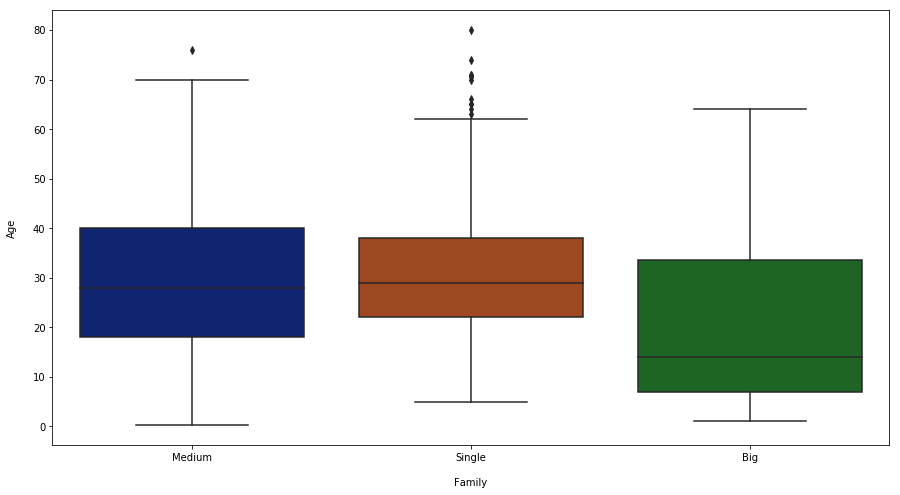plt.figure(figsize=(15,8))
sns.boxplot(x='Title',data=dataset,y='Age', palette = "dark")
plt.xlabel('\nTitle')
plt.ylabel('Age\n')
plt.show()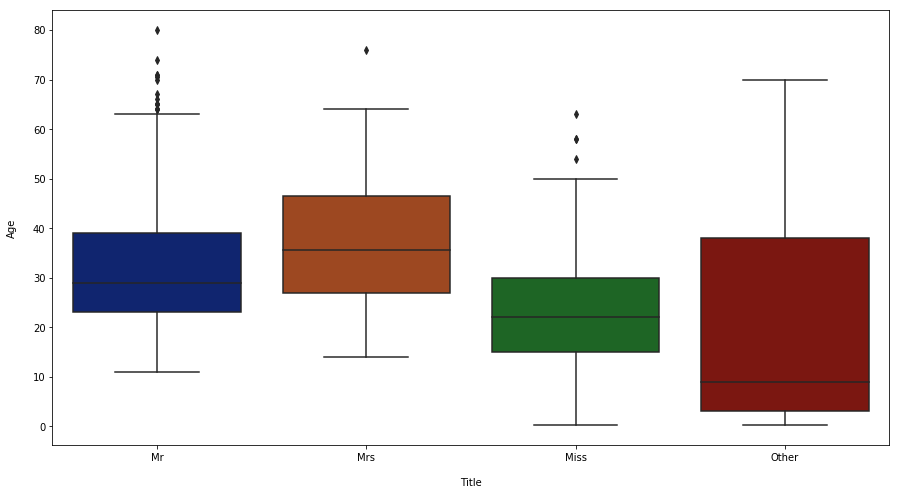plt.figure(figsize=(15,8))
sns.boxplot(x='Ticket',data=dataset,y='Age', palette = "dark")
plt.xlabel('\nTicket')
plt.ylabel('Age\n')
plt.show()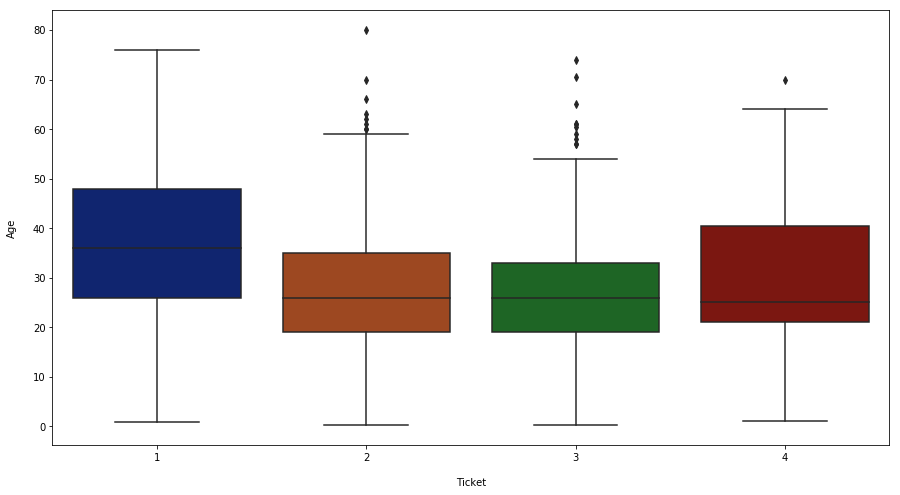plt.figure(figsize=(15,8))
sns.boxplot(x='Sex',data=dataset,y='Age', palette = "dark")
plt.xlabel('\nSex')
plt.ylabel('Age\n')
plt.show()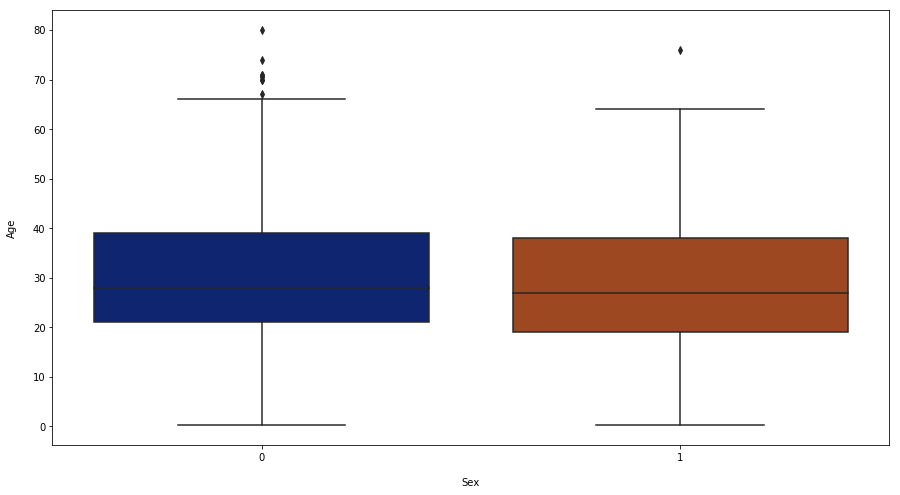plt.figure(figsize=(15,8))
sns.boxplot(x='Fare',data=dataset,y='Age', palette = "dark")
plt.xlabel('\nFare')
plt.ylabel('Age\n')
plt.show()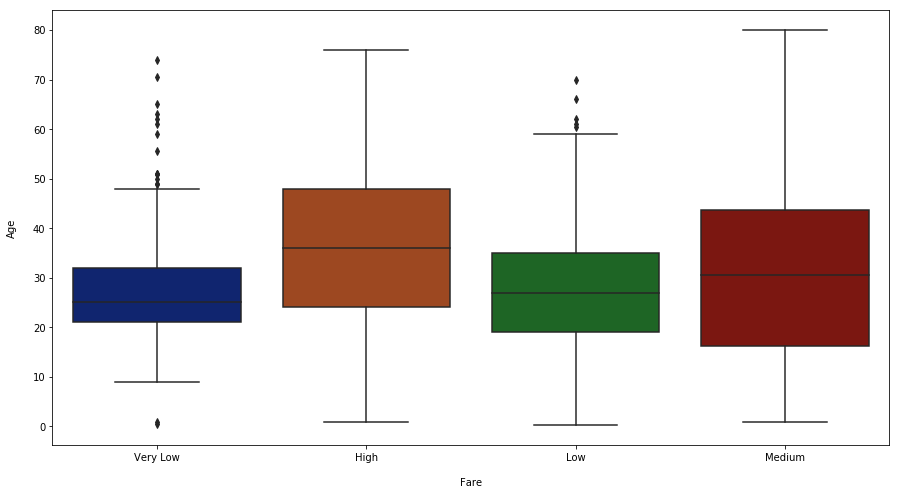plt.figure(figsize=(15,8))
sns.boxplot(x='Embarked',data=dataset,y='Age', palette = "dark")
plt.xlabel('\nEmbarked')
plt.ylabel('Age\n')
plt.show()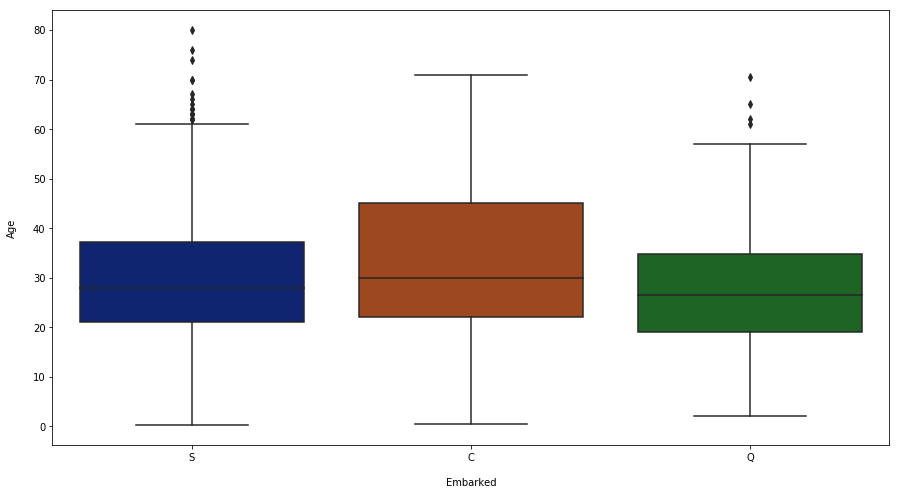plt.figure(figsize=(15,8))
sns.boxplot(x='Pclass',data=dataset,y='Age', palette = "dark")
plt.xlabel('\nPclass')
plt.ylabel('Age\n')
plt.show()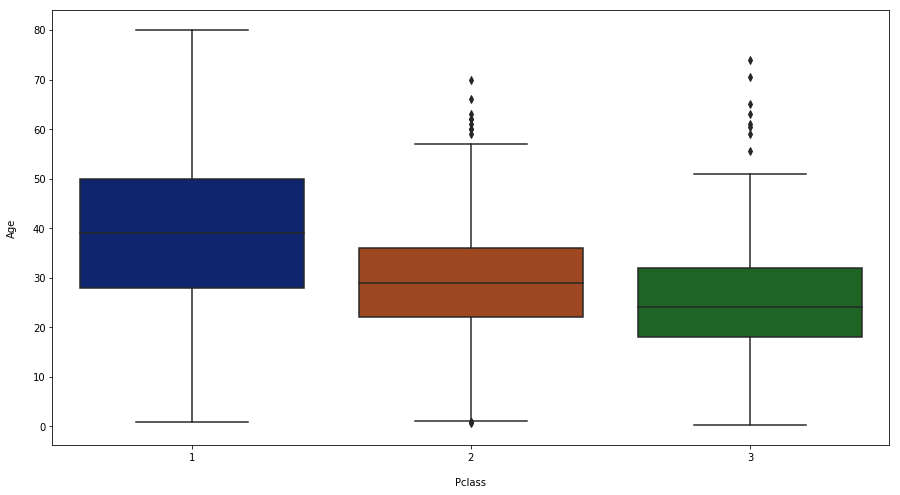plt.figure(figsize=(15,8))
sns.boxplot(x='Cabin',data=dataset,y='Age', palette = "dark")
plt.xlabel('\nCabin')
plt.ylabel('Age\n')
plt.show()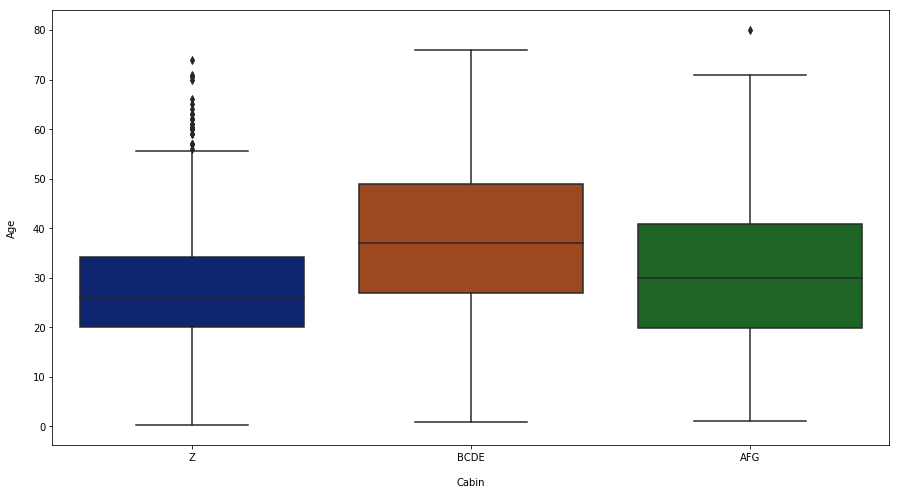With the boxplots results we can say that Pclass and Title are important to calculate the age.

So we can calculate the median of the age grouped by these features.

medians = pd.DataFrame(dataset.groupby(['Pclass', 'Title'])['Age'].median())
medians

Age
Pclass Title
1 Miss 30.0
Mr 41.5
Mrs 45.0
Other 42.0
2 Miss 20.0
Mr 30.0
Mrs 30.5
Other 15.5
3 Miss 18.0
Mr 26.0
Mrs 31.0
Other 6.0

Let's separate the dataset indexes that have Age nan values.

By creating list of medians based on variables values it's possible to replace nan's safely.

ages = []
for i in dataset[dataset.Age.isnull() == True][["Pclass","Title"]].values:
ages.append(medians.ix[(i,  i)].Age)

dataset.Age[dataset.Age.isnull() == True] = ages


Doing the same for Train and Test.

index = dataset[dataset.Age.isnull() == True].index
train_idx = index[index <= 890]
test_idx = index[index > 890]

train['Age'][train.index.isin(train_idx)] = dataset['Age'][dataset.index.isin(train_idx)].values
test['Age'][test.index.isin(test_idx - 891)] = dataset['Age'][dataset.index.isin(test_idx)].values


Now that we have all ages, it's easy to group them into categories.

ages = []
for i in dataset.Age:
if i < 18:
ages.append('less_18')
elif i >= 18 and i < 50:
ages.append('18_50')
else:
ages.append('greater_50')

dataset.Age = ages

ages = []
for i in train.Age:
if i < 18:
ages.append('less_18')
elif i >= 18 and i < 50:
ages.append('18_50')
else:
ages.append('greater_50')

train.Age = ages

ages = []
for i in test.Age:
if i < 18:
ages.append('less_18')
elif i >= 18 and i < 50:
ages.append('18_50')
else:
ages.append('greater_50')

test.Age = ages


Let's now see the probabilities for each category.

sns.factorplot(x='Age',
size= 7,
aspect= 2,
data=train,
y ='Survived',
palette = "dark")
plt.xlabel('\nAge')
plt.ylabel('Survival Probability\n')
plt.show()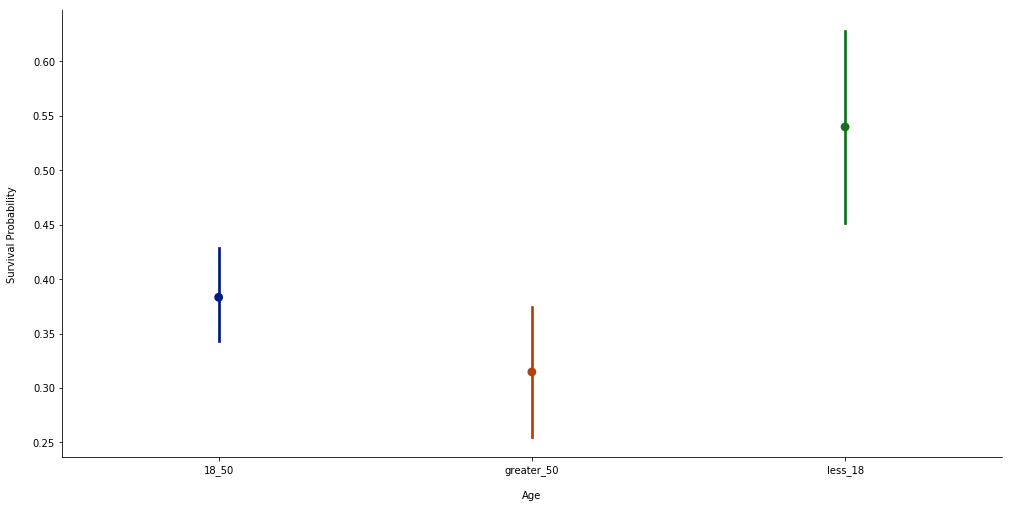Children and teenagers have more probability to survive.

### Splitting variables into train and test sets

# PassengerId is not relevant and Sex (we don't want this variable to get dummied - see next cell)
x_train = train.loc[:, ~train.columns.isin(['PassengerId', 'Survived', 'Sex'])]
y_train = train.Survived
x_test = test.loc[:, ~test.columns.isin(['PassengerId', 'Survived', 'Sex'])]


Transforming each category of each column into another column with 1 or 0 value (get_dummies).

x_train = pd.get_dummies(x_train)
x_train["Sex"] = train.Sex # adding sex
x_test = pd.get_dummies(x_test)
x_test["Sex"] = test.Sex # adding sex


# 2-Features Importance

Lets see the most important features with the Random Forest Classifier.

rf = RandomForestClassifier()
rf.fit(x_train, y_train)

RandomForestClassifier(bootstrap=True, class_weight=None, criterion='gini',
max_depth=None, max_features='auto', max_leaf_nodes=None,
min_impurity_decrease=0.0, min_impurity_split=None,
min_samples_leaf=1, min_samples_split=2,
min_weight_fraction_leaf=0.0, n_estimators=10,
n_jobs=None, oob_score=False, random_state=None,
verbose=0, warm_start=False)
feature_importances = pd.DataFrame(rf.feature_importances_,
index = x_train.columns,
columns=['importance']).sort_values('importance',ascending=False)

plt.figure(figsize=(20,8))
plt.xticks(rotation=45)
plt.plot(feature_importances)
plt.scatter(y=feature_importances.importance,x=feature_importances.index)
plt.ylabel('Importance\n')
plt.grid()
plt.show()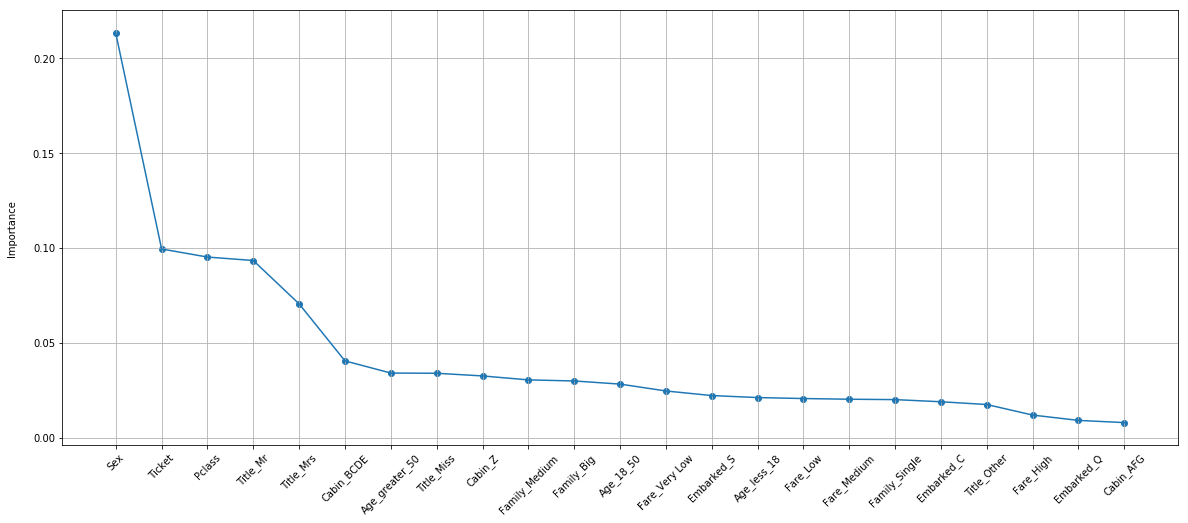It seems that 'Sex' and 'Mr' are the most important variables.

# 3-Train Model

Here we are going to try some models.

ABC = AdaBoostClassifier(DecisionTreeClassifier())

ABC_param_grid = {"base_estimator__criterion" : ["gini", "entropy"],
"base_estimator__splitter" :   ["best", "random"],
"algorithm" : ["SAMME","SAMME.R"],
"n_estimators" :[5,6,7,8,9,10,20],
"learning_rate":  [0.001, 0.01, 0.1, 0.3]}

gsABC = GridSearchCV(ABC, param_grid = ABC_param_grid, cv = 10, scoring = "accuracy", n_jobs = 6, verbose = 1)

gsABC.fit(x_train,y_train)

gsABC.best_score_

Fitting 10 folds for each of 224 candidates, totalling 2240 fits

[Parallel(n_jobs=6)]: Using backend LokyBackend with 6 concurrent workers.
[Parallel(n_jobs=6)]: Done  38 tasks      | elapsed:    7.6s
[Parallel(n_jobs=6)]: Done 188 tasks      | elapsed:   12.2s
[Parallel(n_jobs=6)]: Done 438 tasks      | elapsed:   18.6s
[Parallel(n_jobs=6)]: Done 788 tasks      | elapsed:   27.8s
[Parallel(n_jobs=6)]: Done 1238 tasks      | elapsed:   40.8s
[Parallel(n_jobs=6)]: Done 1788 tasks      | elapsed:   55.6s
[Parallel(n_jobs=6)]: Done 2240 out of 2240 | elapsed:  1.1min finished

0.8170594837261503

### ExtraTress

ExtC = ExtraTreesClassifier()

ex_param_grid = {"max_depth": [3, 4, 5],
"max_features": [3, 10, 15],
"min_samples_split": [2, 3, 4],
"min_samples_leaf": [1, 2],
"bootstrap": [False,True],
"n_estimators" :[100,200,300],
"criterion": ["gini","entropy"]}

gsExtC = GridSearchCV(ExtC, param_grid = ex_param_grid, cv = 10, scoring = "accuracy", n_jobs = 6, verbose = 1)

gsExtC.fit(x_train,y_train)

ext_best = gsExtC.best_estimator_

gsExtC.best_score_

[Parallel(n_jobs=6)]: Using backend LokyBackend with 6 concurrent workers.

Fitting 10 folds for each of 648 candidates, totalling 6480 fits

[Parallel(n_jobs=6)]: Done  38 tasks      | elapsed:    6.2s
[Parallel(n_jobs=6)]: Done 188 tasks      | elapsed:   31.6s
[Parallel(n_jobs=6)]: Done 438 tasks      | elapsed:  1.3min
[Parallel(n_jobs=6)]: Done 788 tasks      | elapsed:  2.3min
[Parallel(n_jobs=6)]: Done 1238 tasks      | elapsed:  3.8min
[Parallel(n_jobs=6)]: Done 1788 tasks      | elapsed:  5.6min
[Parallel(n_jobs=6)]: Done 2438 tasks      | elapsed:  7.6min
[Parallel(n_jobs=6)]: Done 3188 tasks      | elapsed: 10.1min
[Parallel(n_jobs=6)]: Done 4038 tasks      | elapsed: 12.7min
[Parallel(n_jobs=6)]: Done 4988 tasks      | elapsed: 15.7min
[Parallel(n_jobs=6)]: Done 6038 tasks      | elapsed: 19.0min
[Parallel(n_jobs=6)]: Done 6480 out of 6480 | elapsed: 20.5min finished

0.8338945005611672

### Random Forest

rf_test = {"max_depth": [24,26],
"max_features": [6,8,10],
"min_samples_split": [3,4],
"min_samples_leaf": [3,4],
"bootstrap": [True],
"n_estimators" :[50,80],
"criterion": ["gini","entropy"],
"max_leaf_nodes":[26,28],
"min_impurity_decrease":[0.0],
"min_weight_fraction_leaf":[0.0]}

tuning = GridSearchCV(estimator = RandomForestClassifier(), param_grid = rf_test, scoring = 'accuracy', n_jobs = 6, cv = 10)

tuning.fit(x_train,np.ravel(y_train))

rf_best = tuning.best_estimator_

tuning.best_score_

0.8338945005611672

### GBM

GBM = GradientBoostingClassifier()

gb_param_grid = {'loss' : ["deviance"],
'n_estimators' : [450,460,500],
'learning_rate': [0.1,0.11],
'max_depth': [7,8],
'min_samples_leaf': [30,40],
'max_features': [0.1,0.4,0.6]}

gsGBC = GridSearchCV(GBM, param_grid = gb_param_grid, cv = 10, scoring = "accuracy", n_jobs = 6, verbose = 1)

gsGBC.fit(x_train,y_train)

gbm_best = gsGBC.best_estimator_

gsGBC.best_score_

Fitting 10 folds for each of 72 candidates, totalling 720 fits

[Parallel(n_jobs=6)]: Using backend LokyBackend with 6 concurrent workers.
[Parallel(n_jobs=6)]: Done  38 tasks      | elapsed:   12.1s
[Parallel(n_jobs=6)]: Done 188 tasks      | elapsed:  1.3min
[Parallel(n_jobs=6)]: Done 438 tasks      | elapsed:  3.0min
[Parallel(n_jobs=6)]: Done 720 out of 720 | elapsed:  5.1min finished

0.8361391694725028

### SVC

SVMC = SVC(probability=True)

svc_param_grid = {'kernel': ['rbf'],
'gamma': [0.027,0.029,0.03,0.031],
'C': [45,55,76,77,78,85,95,100],
'tol':[0.001,0.0008,0.0009,0.0011]}

gsSVMC = GridSearchCV(SVMC, param_grid = svc_param_grid, cv = 10, scoring = "accuracy", n_jobs = 6, verbose = 1)

gsSVMC.fit(x_train,y_train)

svm_best = gsSVMC.best_estimator_

gsSVMC.best_score_

Fitting 10 folds for each of 128 candidates, totalling 1280 fits

[Parallel(n_jobs=6)]: Using backend LokyBackend with 6 concurrent workers.
[Parallel(n_jobs=6)]: Done  38 tasks      | elapsed:    5.5s
[Parallel(n_jobs=6)]: Done 188 tasks      | elapsed:   26.7s
[Parallel(n_jobs=6)]: Done 438 tasks      | elapsed:  1.1min
[Parallel(n_jobs=6)]: Done 788 tasks      | elapsed:  2.1min
[Parallel(n_jobs=6)]: Done 1238 tasks      | elapsed:  3.4min
[Parallel(n_jobs=6)]: Done 1280 out of 1280 | elapsed:  3.6min finished

0.8148148148148148

### XGBoost

XGB = XGBClassifier()

xgb_param_grid = {'learning_rate': [0.1,0.04,0.01],
'max_depth': [5,6,7],
'n_estimators': [350,400,450,2000],
'gamma': [0,1,5,8],
'subsample': [0.8,0.95,1.0]}

gsXBC = GridSearchCV(XGB, param_grid = xgb_param_grid, cv = 10, scoring = "accuracy", n_jobs = 6, verbose = 1)

gsXBC.fit(x_train,y_train)

xgb_best = gsXBC.best_estimator_

gsXBC.best_score_

Fitting 10 folds for each of 432 candidates, totalling 4320 fits

[Parallel(n_jobs=6)]: Using backend LokyBackend with 6 concurrent workers.
[Parallel(n_jobs=6)]: Done  38 tasks      | elapsed:   25.1s
[Parallel(n_jobs=6)]: Done 188 tasks      | elapsed:  2.1min
[Parallel(n_jobs=6)]: Done 438 tasks      | elapsed:  5.7min
[Parallel(n_jobs=6)]: Done 788 tasks      | elapsed: 10.8min
[Parallel(n_jobs=6)]: Done 1238 tasks      | elapsed: 17.2min
[Parallel(n_jobs=6)]: Done 1788 tasks      | elapsed: 25.1min
[Parallel(n_jobs=6)]: Done 2438 tasks      | elapsed: 34.2min
[Parallel(n_jobs=6)]: Done 3188 tasks      | elapsed: 44.8min
[Parallel(n_jobs=6)]: Done 4038 tasks      | elapsed: 57.1min
[Parallel(n_jobs=6)]: Done 4320 out of 4320 | elapsed: 61.8min finished

0.8294051627384961

## Models Correlations

This is a correlation between the models predictions. With the results we can see if its possible to combine them into a Voting Classifier.

corr = pd.concat([pd.Series(rf_best.predict(x_test), name="RF"),
pd.Series(ext_best.predict(x_test), name="EXT"),
pd.Series(svm_best.predict(x_test), name="SVC"),
pd.Series(gbm_best.predict(x_test), name="GBM"),
pd.Series(xgb_best.predict(x_test), name="XGB"),

plt.figure(figsize=(18,18))
sns.heatmap(corr.corr(),annot=True)
plt.show()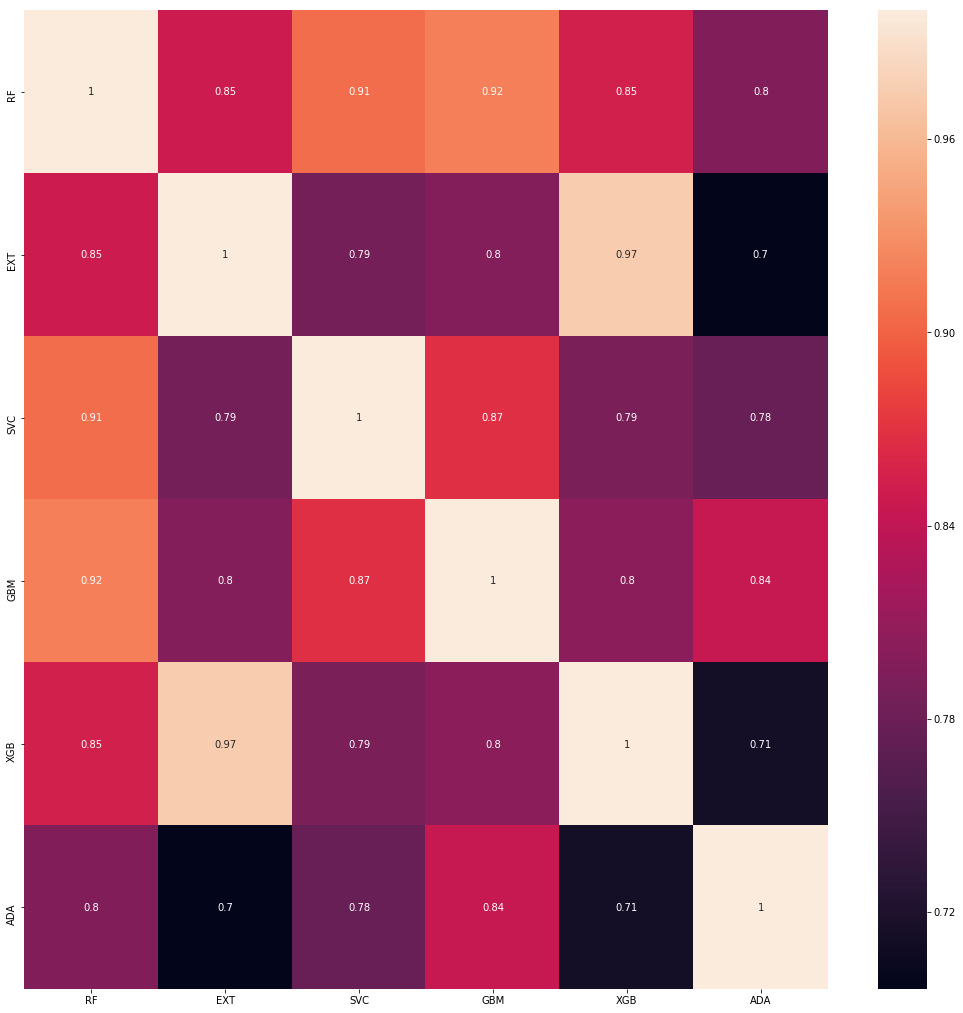# 4-Voting Classifier

We can use a Voting Classifier to ensemble all the models and to build a powerfull one.

voting = VotingClassifier(estimators=[('rfc', rf_best),
('extc', ext_best),
('svc', svm_best),
('gbc',gbm_best),
('xgbc',xgb_best),

v_param_grid = {'voting':['soft',
'hard']} # tuning voting parameter

gsV = GridSearchCV(voting,
param_grid =
v_param_grid,
cv = 10,
scoring = "accuracy",
n_jobs = 6,
verbose = 1)

gsV.fit(x_train,y_train)

v_best = gsV.best_estimator_

gsV.best_score_

Fitting 10 folds for each of 2 candidates, totalling 20 fits

[Parallel(n_jobs=6)]: Using backend LokyBackend with 6 concurrent workers.
[Parallel(n_jobs=6)]: Done  20 out of  20 | elapsed:   20.3s finished

0.8215488215488216

# 5-Submission

Finally, it's time to test the model in the test set and make the submission.

pred = v_best.predict(x_test)

submission = pd.DataFrame(test.PassengerId)
submission["Survived"] = pd.Series(pred)

submission.to_csv("submission.csv",index=False)